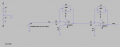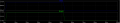# Biased volate resistor logic value in LTSPICE

#### yef smith

Joined Aug 2, 2020
606
Hello,I have built the following circuit ,however i am not sure about resistors R6 and R5.
they are supposed to be bias point setting.
I see that in case my input is 0V my DC at the output is 48mv instead of 0V
what is the mathematiclac logic behind setting properly R6 and R5?
Thanks.#### Ian0

Joined Aug 7, 2020
8,983
Two possibilities:
1. Offset voltage: 1mV offset voltage* gets multiplied by the gain. Then the output offset from the first stage gets multiplied by the gain of the second stage.
2. Input bias current*: 400nA will flow into or out of the input pins. This will create a voltage across the gain-setting resistors, which gets amplified. To eliminate effects of input bias current the resistances on each input should be identical. i.e. R6 should be equivalent to the parallel combination of R3 and R4 = 2.9k (but it does nothing about the offset voltages)

* See page 3 of the datasheet.